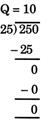Courses

# Test: Division - 3

## 20 Questions MCQ Test Mathematics (Maths) for Class 3 | Test: Division - 3

Description
This mock test of Test: Division - 3 for Class 3 helps you for every Class 3 entrance exam. This contains 20 Multiple Choice Questions for Class 3 Test: Division - 3 (mcq) to study with solutions a complete question bank. The solved questions answers in this Test: Division - 3 quiz give you a good mix of easy questions and tough questions. Class 3 students definitely take this Test: Division - 3 exercise for a better result in the exam. You can find other Test: Division - 3 extra questions, long questions & short questions for Class 3 on EduRev as well by searching above.
QUESTION: 1

### (27 ÷ 3) × 8 = ______

Solution:

(27 ÷ 3) × 8 = 9 × 8 = 72

QUESTION: 2

### A number with "0" at one's place is divisible by:

Solution:

A number having 0 at one?s place is divisible by 2, 5 and 10.

QUESTION: 3

### How many 8's are there in 120?

Solution:

120 ÷ 8 = 15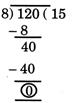QUESTION: 4

The least number among the following is:

Solution:

68 ÷ 4 = 17, 68 + 4 = 72, 68 × 4 = 272, 68 − 4 = 64 Here, 17 is the least.

QUESTION: 5

Anupama divided 498 by 8 and obtained a quotient of 62 and remainder 2. For this problem, which of these checks will help Anupama to find out her mistakes?

Solution:

In division problems, remainder should always be less than divisor.

QUESTION: 6

0 ∗ 18 = 0 This will be true if * is replaced by:

Solution:

0 × 18 = 0
0 ÷ 18 = 0

QUESTION: 7

If we start subtracting 4 from 19 stepwise as shown, we will be able to subtract 4 times.If we start subtracting 4 from 169 in a similar manner, how many times will we be able to subtract?

Solution: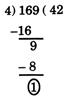QUESTION: 8

Ramya divides a 3-digit number by 10. What can we say about the quotient?

Solution:

The quotient is obtained by removing one?s digit
Ex: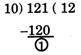By removing one?s digit of 121 we get 12.

QUESTION: 9

This is a seating arrangement for students in a classroom with each box showing one chair. Thy chairs which have a star mark are occupied.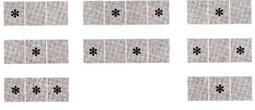Which of the following statement is true?

Solution:

Number of empty chairs = Number of occupied chairs = 13

QUESTION: 10

If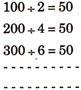then 800 ÷ 16 = _______.

Solution:

Observing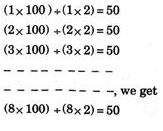QUESTION: 11

Raju has four plates of laddoos. He wants to put them equally in four plates. What should be the number of laddoos in each plate?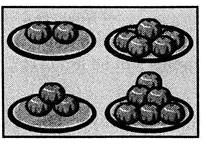Solution:

Total number of laddoos = 2 + 5 + 3 + 6 = 16
∴ Number of laddoos in each plate = 16/4 = 4

QUESTION: 12

There are 21 bananas.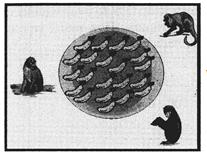What is the equal share of each monkey?

Solution:

Share of each monkey = 21/3 = 7

QUESTION: 13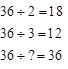Missing number is:

Solution:

36 + 1 = 36

QUESTION: 14

There are few pencils in a box. Rahul took out those pencils from the box and put them in five groups of each 4. Three pencils were left in the box. How many pencils were there in the box?

Solution:

Number of pencils he took out from the box = 5 × 4 = 20
Remaining pencils in the box = 3
∴ Total number of pencils in the box = 20 + 3 = 23

QUESTION: 15

Missing number in the following is: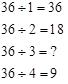Solution:

36 ÷ 3 = 12

QUESTION: 16

A shopkeeper packed 360 biscuits in 12 boxes. What is the number of biscuits in each box?

Solution:

Number of biscuits in each box = 360 ÷ 12 = 30

QUESTION: 17

If this pattern continues, then what is the next number? 80, 40, 20,  ? −−−−−−−

Solution:

Observe the pattern.

QUESTION: 18

245 candles are to be arranged equally in 15 rows. How many candles will be left?

Solution: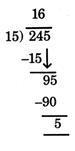5 candles will be left.

QUESTION: 19

If largest 3-digit number is divided by smallest 2-digit number, then:

Solution: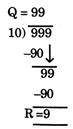QUESTION: 20

If 5 tens are subtracted from 300 and divided by 25, then quotient is:

Solution:

5 tens = 50, 3 hundreds = 300 difference = 300 - 50 = 250